• 文章目录不可约多项式例1因式分解及唯一性定理例 2重因式微商例 3小结参考资料 不可约多项式 定义\large\color{magenta}{\boxed{\color{brown}{定义} }}定义​设 f(x)∈F[x],deg⁡f(x)>0.f(x) \in F[x], \...
文章目录不可约多项式例1因式分解及唯一性定理例 2重因式微商例 3小结参考资料

多项式01——一元多项式和运算
多项式02——整除
多项式03——最大公因式与互素
多项式04——标准分解式
多项式05——多项式函数
多项式07——有理系数和整系数多项式

不可约多项式
$\large\color{magenta}{\boxed{\color{brown}{定义} }}$设 $f(x) \in F[x], \operatorname{deg} f(x)>0 .$ 若存在
$g(x), h(x) \in F[x], \text { 使得 } f(x)=g(x) h(x)$
其中 $\operatorname{deg} g(x)<\operatorname{deg} f(x)$ 且 $\operatorname{deg} h(x)<\operatorname{deg} f(x)$ .则称 $f(x)$ 在F上可约, 否则 $f(x)$ 为不可约多项式.
$\Large\color{violet}{注 :}$多项式的不可约与数域有关;一次多项式都是不可约多项式。
$\large\color{magenta}{\boxed{\color{brown}{命题1} }}$ 若 $p(x)$ 是不可约多项式，则只有c $\mid p(x)$ 和 $c p(x) \mid p(x)$,其中 $c \in \boldsymbol{F}, \quad$ 且 $c \neq 0$
$\large\color{magenta}{\boxed{\color{brown}{命题2} }}$ 设 $f(x), p(x) \in F[x]$ 且 $p(x)$ 是不可约多项式,则 $p(x) \mid f(x)$ 或 $(p(x), f(x))=1 .$
【证明】设 $(p(x), f(x))=d(x),$ 则 $d(x) \mid p(x) .$
又因为 $p(x)$ 是不可约多项式,所以 $d(x)=1$ 或 $d(x) = cp(x)$.
这里 $c^{-1}$ 为 $p(x)$ 的首项系数.
若 $d(x)=c p(x),$ 则 $p(x) \mid f(x)$
$\large\color{magenta}{\boxed{\color{brown}{性质} }}$ 设 $p(x)$ 为数域 $\boldsymbol{F}$ 上的次数大于零的多项式。若 $p(x)$ 对任意 多项式 $f(x)$ 有 $p(x) \mid f(x)$ 或 $(p(x), f(x))=1,$ 则 $p(x)$ 是数域 $\boldsymbol{F}$ 上的不 可约多项式。
$\large\color{magenta}{\boxed{\color{brown}{命题3} }}$ 设 $p(x), f(x), g(x) \in F[x], p(x)$ 是不可约多项式, 且 $p(x) \mid f(x) g(x),$ 则或 $p(x) \mid f(x)$
或 $p(x) \mid g(x)$.
【证明】若 $p(x)$ 不整除 $f(x),$ 因为 $p(x)$ 不可约,则 $(f(x), p(x))=1$
又因为 $p(x) \mid f(x) g(x)$,由互素多项式的性质知 $p(x) \mid g(x) .$
$\large\color{magenta}{\boxed{\color{brown}{性质} }}$ 设 $p(x)$ 为数域 $\boldsymbol{F}$ 上的次数大于零的多项式。若对任意两个多项式 $f(x)$ 和 $g(x),$ 当 $p(x) \mid f(x) g(x)$ 时必有 $p(x) \mid f(x)$ 或者 $p(x) \mid g(x),$ 则 $p(x)$ 一定是数域 $\boldsymbol{F}$ 上的不可约多项式。
$\large\color{magenta}{\boxed{\color{brown}{推论1} }}$ 若 $p(x)$ 是不可约多项式, 且 $p(x) \mid f_{1}(x) f_{2}(x) \ldots f_{s}(x),$ 则对某个 $f_{i}(x), 1 \leq i \leq s,$ 有 $p(x) \mid f_{i}(x)$ 。
例1
设 $f(x) \in F[x]$ 且deg $f(x)=n(n>0), a \in F .$ 令$y=x-a,$ 得 $g(y)=f(y+a) .$ 证明: $f(x)$ 在 $F$ 上可约的充分必要条件是 $g(y)$ 在 $F$ 上可约.
【证明 】必要性. 设 $f(x)$ 次数为 $n .$ 因 $f(x)$ 在 $F$ 上可约，故存在$F$上次数小于n的多项式 $u(x), v(x),$ 使得$f(x)=u(x) v(x) .$ 把 $x$ 用 $y+a$ 替换, 得 $g(y)=s(y) t(y),$其中 $s(y)=u(y+a), t(y)=v(y+a)$ 。
设 $f(x)$ 的首项为 $a_{n} x^{n},$ 简单计算知 $g(y)$ 的首项是 $a_{n} y^{n},$ 这说明 $\operatorname{deg} g(y)=\operatorname{deg} f(x)=n$
同理可得 $\operatorname{deg} s(y)=\operatorname{deg} u(x) ，$\operatorname{deg} t(y)=\operatorname{deg} v(x) 这就证明了 $g(y)$ 在 $F$ 上也可约.
充分性类似可得.
因式分解及唯一性定理
$\large\color{magenta}{\boxed{\color{brown}{定理1} }}$  设 $f(x) \in F[x], \operatorname{deg} f(x) \geq 1,$ 则多项式 $f(x)$:
(1) $f(x)=p_{1}(x) p_{2}(x) \cdots p_{s}(x),$ 其中 $p_{i}(x)$ 为 $F$ 上的不可约多项式 $(i=1,2, \cdots, s) ;$ (存在性定理)
若： $f(x)=p_{1}(x) p_{2}(x) \cdots p_{s}(x),$若不记零次多项式的差异和因式的排列次序，那么$f (x)$分解成不可约因式的乘积的分解式是唯一的。(唯一性定理)
即若有两个分解式
(2) $f(x)=p_{1}(x) p_{2}(x) \cdots p_{s}(x)=q_{1}(x) q_{2}(x) \cdots q_{t}(x)$，其中 $p_{i}(x)$ 与 $q_{j}(x)$ 为 $F$ 上的不可约多项式$(i=1,2, \cdots, s ; j=1,2, \cdots, t),$ 则 $s=t,$ 且经过适当调换因式的顺序后有 $p_{i}(x)$ 与 $q_{i}(x)$ 相伴 $(i=1,2, \cdots, s)$.
相伴 : $p_{i}(x)=cq_{i}(x)$
$\large\color{magenta}{\boxed{\color{brown}{定理2} }}$ 对于任意次数大于零的多项式有如下的标准分解式:
$f(x)=c p_{1}^{e_{1}}(x) p_{2}^{e_{2}}(x) \cdots p_{m}^{e_{m}}(x)$
其中 $p_{i}(x)$ 是首一的两两旦素的不可约多项式$e_{i} \geq 1(i=1,2, \cdots, m)$ ,c 是$f(x)$是首项系数
例 2
设 $f(x)=c p_{1}^{a_{1}}(x) \cdots p_{m}^{a_{m}}(x), g(x)=d p_{1}^{b_{1}}(x) \cdots p_{m}^{b_{m}}(x)$，其中 $p_{i}(x)$ 为首一的两两互素的不可约多项式,对任意的 $i(1 \leq i \leq m),$ 都有 $a_{i} \geq \mathbf{0}, b_{i} \geq 0,$ 且 $a_{i}+b_{i}>0,$ 则
(1) $f(x) \mid g(x)$ 的充要条件是 $a_{i} \leq b_{i}(i=1,2, \cdots, m)$  。
(2) $(f(x), g(x))=p_{1}^{c_{1}}(x) p_{2}^{c_{2}}(x) \cdots p_{m}^{c_{m}}(x),$ 其中$c_{i}=\min \left\{a_{i}, b_{i}\right\}(i=1,2, \cdots, m)$。
$(3)[f(x), g(x)]=p_{1}^{d_{1}}(x) p_{2}^{d_{2}}(x) \cdots p_{m}^{d_{m}}(x),$ 其中$\boldsymbol{d}_{i}=\max \left\{\boldsymbol{a}_{i}, \boldsymbol{b}_{i}\right\}(\boldsymbol{i}=\mathbf{1}, \boldsymbol{2}, \cdots, \boldsymbol{m})$
(4) $(f(x), g(x))[f(x), g(x)]=c^{-1} d^{-1} f(x) g(x),$ 其中 $c, d$ 分别是 $f(x), g(x)$ 的首项系数.
重因式
$\large\color{magenta}{\boxed{\color{brown}{定义} }}$ 设 $f(x), p(x) \in F[x], p(x)$ 是不可约多项式, 若存在$k>1,$ 使得 $p^{k}(x) \mid f(x), p^{k+1}(x)$ 不整除 $f(x),$ 则称 $p(x)$是 $f(x)$ 的 $k$ 重因式.
问题 ：分别在 C上和 $\mathbb{R}$ 上讨论 $p(x)=x^{2}+1$ 是否为$f(x)=\left(x^{4}-1\right)^{3}$ 的重因式.
微商
$\large\color{magenta}{\boxed{\color{brown}{定义} }}$设 $f(x)=a_{n} x^{n}+a_{n-1} x^{n-1}+\cdots+a_{1} x+a_{0}$，定义 $f(x)$ 的导数（一阶微商）为
$f^{\prime}(x)=n a_{n} x^{n-1}+(n-1) a_{n-1} x^{n-2}+\cdots+2 a_{2} x+a_{1}$
例：n次多项式的微商是一个$n-1$次多项式，它的$n$阶微商就 是一个常数，它的n+1阶微商就是零。
直接验证以下性质：
(1) $(f(x)+g(x))^{\prime}=f^{\prime}(x)+g^{\prime}(x)$
(2) $(f(x) g(x))^{\prime}=f^{\prime}(x) g(x)+f(x) g^{\prime}(x)$
(3) $\left(f^{m}(x)\right)^{\prime}=m f^{\prime}(x) f^{m-1}(x)$.
$\large\color{magenta}{\boxed{\color{brown}{定理3} }}$ 设不可约多项式 $p(x)$ 是 $f(x)$ 的 $k$ 重因式 $(k>1),$ 则 $p(x)$ 是 $f^{\prime}(x)$ 的 $k$ -1重因式.
【证明 】由设 $f(x)=p^{k}(x) h(x),$ 其中 $p(x)$ 不整除 $h(x) .$ 直接计算得
$f^{\prime}(x)=k p^{k-1}(x) p^{\prime}(x) h(x)+p^{k}(x) h^{\prime}(x)=p^{k-1}(x)\left(k p^{\prime}(x) h(x)+p(x) h^{\prime}(x)\right)$
显然 $p^{k-1}(x) \mid f^{\prime}(x),$ 但 $p(x)$ 不整除 $k p^{\prime}(x) h(x)+p(x) h^{\prime}(x) .$ 否则有 $p(x)$整除 $p^{\prime}(x) h(x) .$ 而 $p(x)$ 不可约且 $p(x)$ 不整除 $h(x),$ 所以 $p(x)$ 整除 $p^{\prime}(x),$ 这是不可能的, 因为 $p^{\prime}(x)$ 非零, 且次数小于 $p(x)$ 的次数.
$\large\color{magenta}{\boxed{\color{brown}{推论} }}$ 若不可约多项式 $p(x)$ 是 $f(x)$ 的 $k$ 重因式
$(k \geq 1), \text { 则 } p(x) \text { 是 } f^{\prime}(x), f^{\prime \prime}(x), \cdots, f^{(k-1)}(x)$
的因式, 但不是 $f^{(k)}(x)$ 的因式.
$\large\color{magenta}{\boxed{\color{brown}{推论} }}$ 不可约多项式 $p(x)$ 是 $f(x)$ 的重因式的当且仅当 $p(x)$ 是$f(x)$ 与 $f^{\prime}(x)$ 的公因式。
$\large\color{magenta}{\boxed{\color{brown}{推论} }}$ 不可约多项式 $p(x)$ 是 $f(x)$ 的 $k$ 重因式的充分必要条件是 $p(x)$ 为 $\left(f(x), f^{\prime}(x)\right)$ 的 $k$ -1重因式.
【证明 】若 $p(x)$ 是 $f(x)$ 的 $k$ 重因式, 则必是 $f^{\prime}(x)$ 的 $k$ - 1重因式. 因而是 $\left(f(x), f^{\prime}(x)\right)$ 的 $k$ -1重因式.
反之, 若 $f(x)$ 的不可约因式也是 $f^{\prime}(x)$ 的因式,设 $p(x)$ 是 $f(x)$ 的 $l$ 重因式, 则 $p(x)$ 是 $f^{\prime}(x)$ 的$l-1$重因式. 所以 $l=k .$
$\large\color{magenta}{\boxed{\color{brown}{推论} }}$ $f(x)$ 没有重因式的充分必要条件是
$\left(f(x), f^{\prime}(x)\right)=1$
设 $f(x)$ 的标准分解式为 $f(x)=c p_{1}^{e_{1}}(x) p_{2}^{e_{2}}(x) \cdots p_{s}^{e_{s}}(x)$
\begin{aligned} \text { 则 } f^{\prime}(x)=& e_{1} p_{1}^{\prime}(x) c p_{1}^{e_{1}-1}(x) p_{2}^{e_{2}}(x) \cdots p_{s}^{e_{s}}(x)+\\ &+e_{2} p_{2}^{\prime}(x) c p_{1}^{e_{1}}(x) p_{2}^{e_{2}-1}(x) \cdots p_{s}^{e_{s}}(x)+\\ &+\cdots+e_{s} p_{s}^{\prime}(x) c p_{1}^{e_{1}}(x) p_{2}^{e_{2}}(x) \cdots p_{s}^{e_{s}-1}(x) \end{aligned}
所以 $p_{1}^{e_{1}-1}(x) p_{2}^{e_{2}-1}(x) \cdots p_{s}^{e_{s}-1}(x)$ 是 $f(x)$ 和 $f^{\prime}(x)$ 的公因式.
注意到 $p_{1}^{e_{1}}(x)$ 可整除右项中除第一项外的所有各项,但不能整除第一项, 故 $p_{1}^{\rho_{1}}(x)$ 不整除 $f^{\prime}(x) .$ 同理 $p_{i}^{e_{i}}(x)$不整除 $f^{\prime}(x) .$
所以
$\text { }\left(f(x), f^{\prime}(x)\right)=p_{1}^{e_{1}-1}(x) p_{2}^{e_{2}-1}(x) \cdots p_{s}^{e_{s}-1}(x)$
于是
$\frac{f(x)}{\left(f(x), f^{\prime}(x)\right)}=c p_{1}(x) p_{2}(x) \cdots p_{s}(x)$
这个多项式没有重因式, 且与 $f(x)$ 有相同的不可约因式.
通过这种方法, 可以去掉 $f(x)$ 的因式重数.
例 3
求多项式 $f(x)=x^{3}+p x+q$ 有重因式的条件。
$f^{\prime}(x)=3 x^{2}+p \quad 3 f(x)=f^{\prime}(x) x+2 p x+3 q$
$f^{\prime}(x)=(2 p x+3 q)\left(\frac{3}{2 p} x-\frac{3 a}{4 p^{2}}\right)+\left(p+\frac{27 q^{2}}{4 p^{2}}\right)$
$f(x)=x^{3}+p x+q$ 有重因式，故 $\left(p+\frac{27 q^{2}}{4 p^{2}}\right)=0,4 p^{3}=27 q^{2}$
小结
(1) 不可约多项式
(2) 标准分解式
(3) 重因式
参考资料
高等代数 厦门大学
高等代数，林亚南，高等教育出版社
《高等代数》（第四版） 高等教育出版社
高等代数    电子科技大学
高等代数_安阳师范学院
《高等代数》（第五版）


展开全文• 借助复数域K上n阶矩阵A的Jordon标准形JA,给出A的一种特殊分解式,讨论矩阵多项式空间K[JA]={f(JA)|f(x)∈K[x]}的基及其子空间的直和分解,进而确定复数域上矩阵多项式空间K[A]的一个关于子空间的直和分解式及其一组基....
• 关于行列因子、不变因子、初等因子、smith标准型、Jordan标准型、最小多项式的定义 应用实例 *** 行列因子、不变因子、初等因子、smith标准型、Jordan标准型、最小多项式的matlab实现 ...
版权声明：<–本博客所有内容均为自己在学习工作中的总结、摘录等-- --转载请注明出处-- --如有侵权请联系删除–>	https://blog.csdn.net/ai359005521/article/details/85068305
资源中包含了行列式因子、不变因子、初等因子、smith标准型、Jordan标准型、最小多项式的matlab实现。运行环境为matlab R2017
行列式因子、不变因子、初等因子、smith标准型、Jordan标准型、最小多项式的matlab实现
一下是代码实现
1.求矩阵A的smith标准型、行列式因子、初等因子、不变因子、最小多项式和jordan标准型
%all--得到矩阵A的smith标准型、行列式因子、初等因子、不变因子、最小多项式和jordan标准型
A = input('A = ');        %获取矩阵A
n = size(A,1);            %将矩阵A的行数赋值给n
syms x;                   %声明变量x
E = eye(n);               %E为n阶的单位矩阵
C = x*E-A;                %C为矩阵A的特征矩阵
%1---求lambda矩阵的行列式因子（开始）
h = [];
for k = 1:n
y = [];
%从n个元素（第一个元素到第n个元素的所有元素）中取出k项，其中所有可能的组合放在p中（组合数）
p = nchoosek(1:n,k);   q = p;
m = size(p,1);
%1-b将所有的i阶行列式求出
for i = 1:m
a = p(i,:);
for j = 1:m
b = q(j,:);
B = [];
for s = 1:k
g = [];
for t = 1:k
g = [g,C(a(s),b(t))];
end
B=[B;g];
end
if det(B) ~= 0
y = [y,det(B)];
end
end
end
%1-b结束
o = size(y,2);
%1-a--求所有j阶行列式的最大公因子（开始）
for j = 1:o-1
y(j+1) = gcd(y(j),y(j+1));
end
%1-a--结束
h = [h,y(o)];
end
%1---求lambda矩阵的行列式因子（结束）
d = [];
d = [d,h(1)];
for i = 2:n
%     d = [d,factor(h(i)/h(i-1))];
d = [d,expand(h(i)/h(i-1))];
end
%2---将上面所求行列式因子进行因式分解，得到因式分解后的行列式因子
k = size(h,2);
for i = 1:k
t = h(i);
t = factor(t);
l = size(t,2);
h(i)=1;

h(i) = h(i)*t(j);
end
end
%2---结束
%3---将上面所求不变因子进行因式分解，得到因式分解后的不变因子
k = size(d,2);
for i = 1:k
t = d(i);
t = factor(t);
l = size(t,2);
d(i)=1;
for j = 1:l
d(i) = d(i)*t(j);
end
end
%3---结束
min_poly = d(k);   %由定理知，在A为n*n的矩阵中，A的最小多项式为A的第n个不变因子dn（lambda）
%4---求初等因子（通过不变因子得到初等因子）
%5---将不变因子的每一项分别进行因式分解
%（把每一项中因式分解中相同的元素相乘，不相同的元素分离，分别放在统一数组的不同位置）
u = size(d,2);
y = [];
for r = 1:u
a = d(r);
a = factor(a);
b = unique(a);
n = size(a,2);
m = size(b,2);
c= [];
for i = 1:m
k = 0;
for j = 1:n
if (b(i) == a(j))
k = k+1;
end
end
c = [c,b(i).^k];
end
y = [y,c];
end
%5--结束
%6---将上面不变因子因式分解又组合后的数组中的1剔除，便的到了初等因子
s = size(y,2);
for v = s:-1:1
if y(v) == 1
y(v) = [];
end
end
%6---结束
%4---结束
smith = diag(d);
j = jordan(A);
fprintf("smith标准型为:\n");
smith
fprintf("行列式因子为:\n");
h
fprintf("不变因子为:\n");
d
fprintf("初等因子为:\n");
y
fprintf("最小多项式为:\n");
min_poly
fprintf("jordan标准型为:\n");
j
%all---结束


2.用辗转相除法求两个多项式的最大公因式
%all--用辗转相除法求两个多项式的最大公因式
function g = gcd(a,b)     %a、b分别为两个多项式的系数，g是a、b的最大公因式
for i=1:100
%1--将a和b的最高次数进行比较，将最高次数较高的多项式赋给a
sizei = size(a);
size1 = sizei(2);
sizei = size(b);
size2 = sizei(2);
if(size1<size2)
c = a;
a = b;
b = c;
end
%1--结束
%用多项式a除多项式b，得到商为q，余r
[q,r] = deconv(a,b);
sizei = size(r);
size3 = sizei(2);
if r(size3)~=0
r(r==0)=[];
end
if r == 0
g = b;
break;
end
a = b;
b = r;
end
g = g/g(1,1);
end
%all--结束


运行结果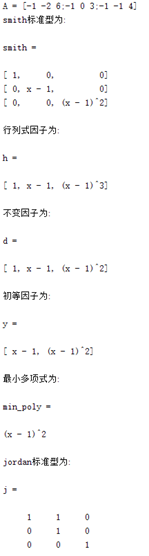展开全文不变因子
• 公因与最大公因 1.公因与最大公因 (1)定义: (2)最大公因的判定: 引理:设f(x),g(x)∈K[x]f(x),g(x)∈K[x]f(x),g(x)∈K[x],如果在K[x]K[x]K[x]中有下述等式成立:f(x)=h(x)g(x)+r(x)(1)f(x)=h(x)g(x)+r(x...
一.公因式与最大公因式(7.3)
1.公因式与最大公因式
(1)定义:注意:$0$是$0$与$0$的最大公因式,由于此时没有次数最高的公因式,故用次数最高作为最大公因式的定义不能包含所有情况,此时最大公因式是所有公因式中次数最小的

(2)最大公因式的存在性与求法:

引理:设$f(x),g(x)∈K[x]$,如果在$K[x]$中有下述等式成立:$f(x)=h(x)g(x)+r(x)\qquad(1)$则$c(x)\,|\,f(x)$且$c(x)\,|\,g(x)⇔c(x)\,|\,g(x)$且$c(x)\,|\,r(x$;从而,$d(x)$是$f(x)$与$g(x)$的最大公因式当且仅当$d(x)$是$g(x)$与$r(x)$的最大公因式定理1:对于$K[x]$中的$∀2$个多项式$f(x),g(x)$,$∃$它们的1个最大公因式$d(x)$,并且$∃u(x),v(x)∈K[x]$,使得$d(x)=u(x)f(x)+v(x)g(x)\qquad(2)$在求$f(x)$与$g(x)$的最大公因式时,利用下述命题可以降低计算量
命题1:设$f(x),g(x)∈K[x],a,b∈K^*$,则$d(x)$是$f(x)$与$g(x)$的1个最大公因式当且仅当$d(x)$是$af(x)$与$bg(x)$的1个最大公因式(3)最大公因式的性质:

命题2:在$K[x]$中,设$d(x)$是$f(x)$与$g(x)$的1个最大公因式,则对于$∀a∈K^*$都有$ad(x)$是$f(x)$与$g(x)$的最大公因式命题3:设$f(x),g(x)\in K[x]$,数域$F\supe K$,则$f(x)$与$g(x)$在$K[x]$中的首一最大公因式等于它们在$F[x]$中的首一最大公因式,即$f(x)$与$g(x)$的首一最大公因式不随数域的扩大而改变2.互素的多项式(1)定义:(2)判定:

定理2:$K[x]$中的2个多项式$f(x),g(x)$互素的充要条件是:$∃u(x),v(x)\in K[x]$,使得$u(x)f(x)+v(x)g(x)=1\qquad(3)$(命题3的)推论1:设$f(x),g(x)\in K[x]$,数域$F\supe K$,则$f(x)$与g(x)在$K[x]$中互素当且仅当$f(x)$与$g(x)$在$F[x]$中互素(3)性质:

性质1:在$K[x]$中,如果$f(x)\,|\,g(x)h(x),(f(x),g(x))=1$那么$f(x)\,|\,h(x)$性质2:在$K[x]$中,如果$f(x)\,|\,h(x),g(x)\,|\,h(x),(f(x),g(x))=1$那么$f(x)g(x)\,|\,h(x)$性质3:在$K[x]$中,如果$(f(x),h(x))=1,(g(x),h(x))=1,$那么$(f(x)g(x),h(x))=1$
可推广为:若$(f_i(x),h(x))=1\,(i=1,2...s)$,则$(f_1(x)f_2(x)...f_s(x),h(x))=1$

3.推广到多个多项式的情况
(1)最大公因式的定义:(2)最大公因式的存在性:

用数学归纳法可以证明:在$K[x]$中,$∀s≥2$个多项式$f_1(x),f_2(x)...f_s(x)$的最大公因式存在(3)互素的定义:(4)互素的判定:

定理3:在$K[x]$中,$f_1(x),f_2(x)...f_s(x)$互素的充要条件是:$∃u_1(x),u_2(x)...u_s(x)∈K[x]$,使得$u_1(x)f_1(x)+u_2(x)f_2(x)+...u_s(x)f_s(x)=1\qquad(8)$
注意:$s≥3$个多项式互素时,它们不一定两两互素,如设$f_1(x)=x+1,f_2(x)=x^2+3x+2,f_3(x)=x-1$则$(f_1(x),f_2(x))=x+1,(f_1(x),f_2(x),f_3(x))=1$,因此$f_1(x),f_2(x),f_3(x)$互素而$f_1(x),f_2(x)$不互素

4.推广到整数环上的情况
(1)最大公约数:定理4:对$∀a,b∈Z$,都存在它们的1个最大公因数$d$,并且$∃u,v∈Z$,使得$ua+vb=d\qquad(9)$(2)互素:定理5:$a,b∈Z$互素当且仅当$∃u,v∈Z$,使得$ua+vb=1\qquad(10)$

互素的整数的性质:
①若$a\,|\,bc$且$(a,b)=1$,则$a\,|\,c$
②若$a\,|\,c,b\,|\,c$且$(a,b)=1$,则$a\,|\,bc$
③若$(a,c)=1,(b,c)=1$,则$(ab,c)=1$
③的推广:(3)最小公倍数:5.$λ-$矩阵的行列式因子(最大公因式的应用之一)
(1)$λ-$矩阵的行列式因子: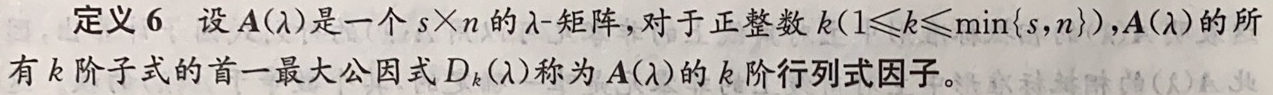定理6:相抵的$λ-$矩阵,它们的秩相等,并且各阶行列式因子也对应相等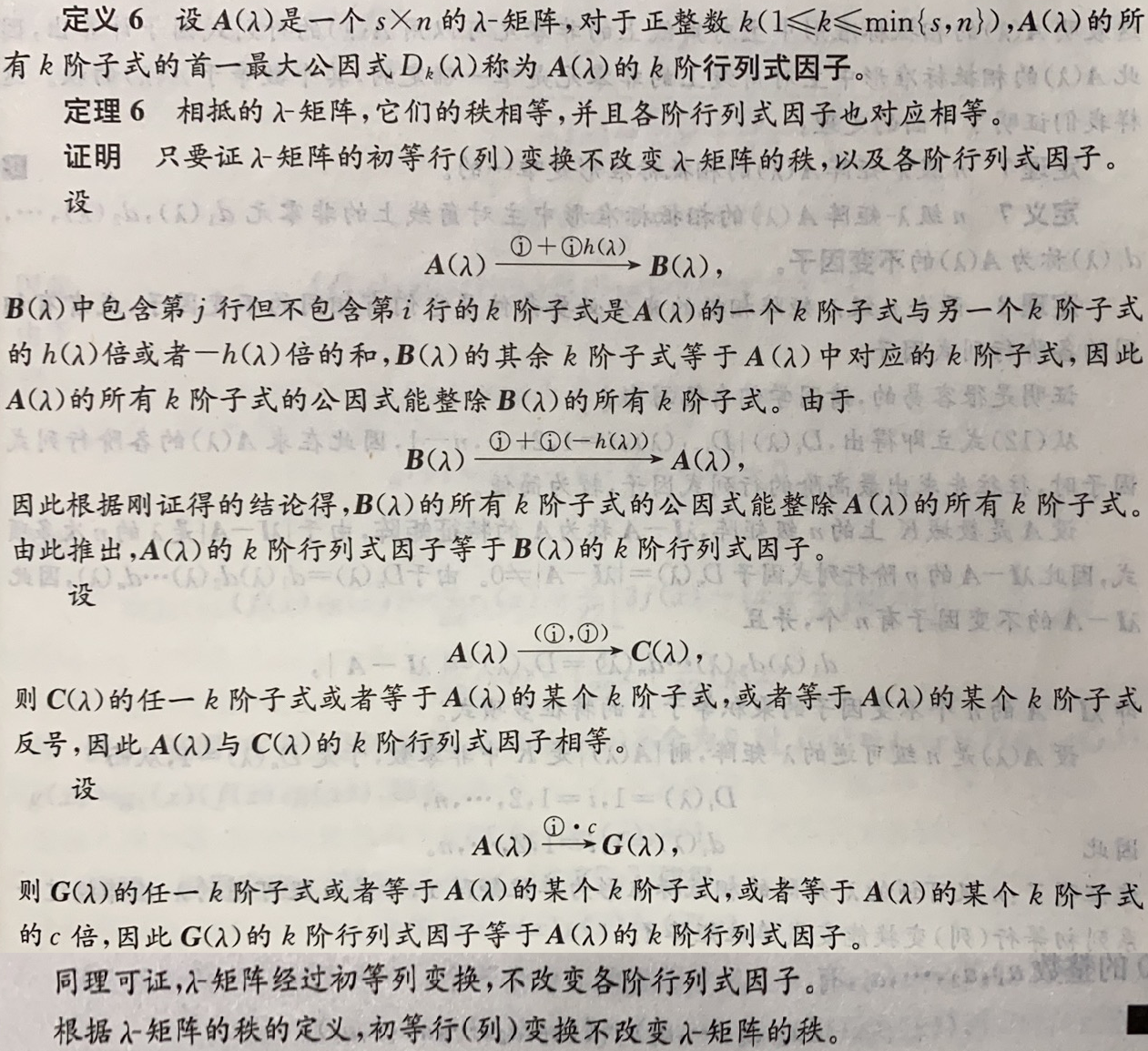(2)相抵标准形的唯一性::

定理7:$n$级$λ-$矩阵$A(λ)$的相抵标准形是唯一的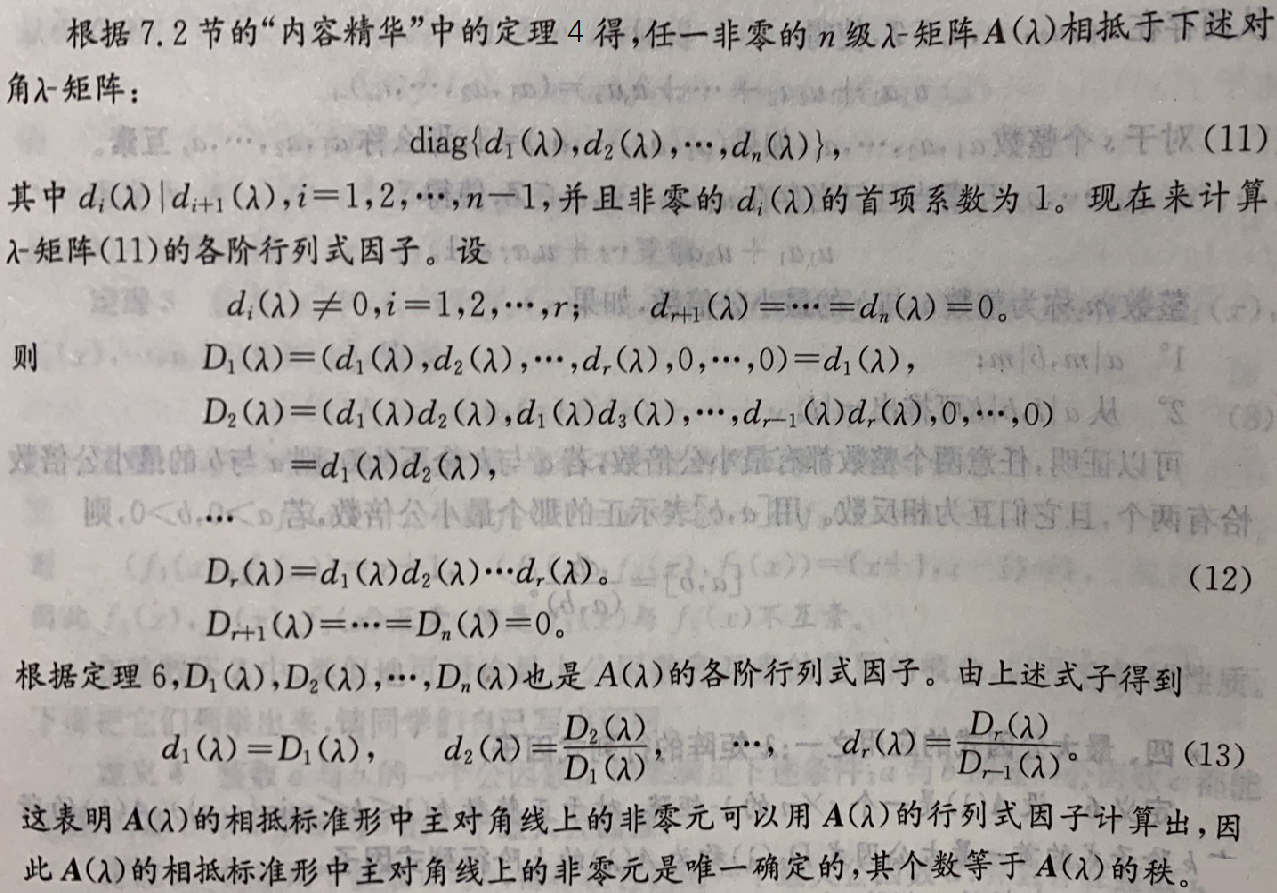(3)不变因子: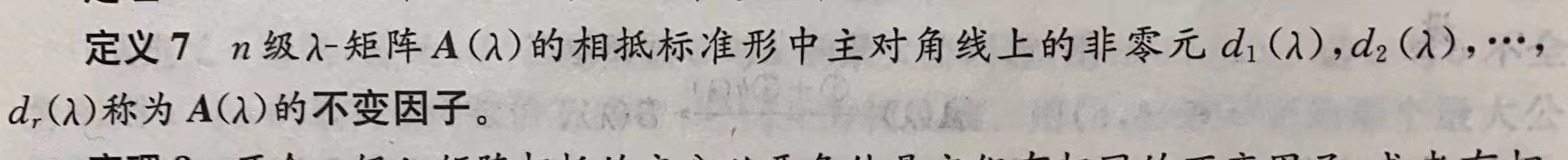定理8:2个$n$级$λ-$矩阵相抵的充要条件是它们有相同的不变因子,或者有相同的各阶行列式因子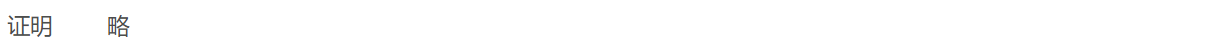(4)可逆与相抵的判定: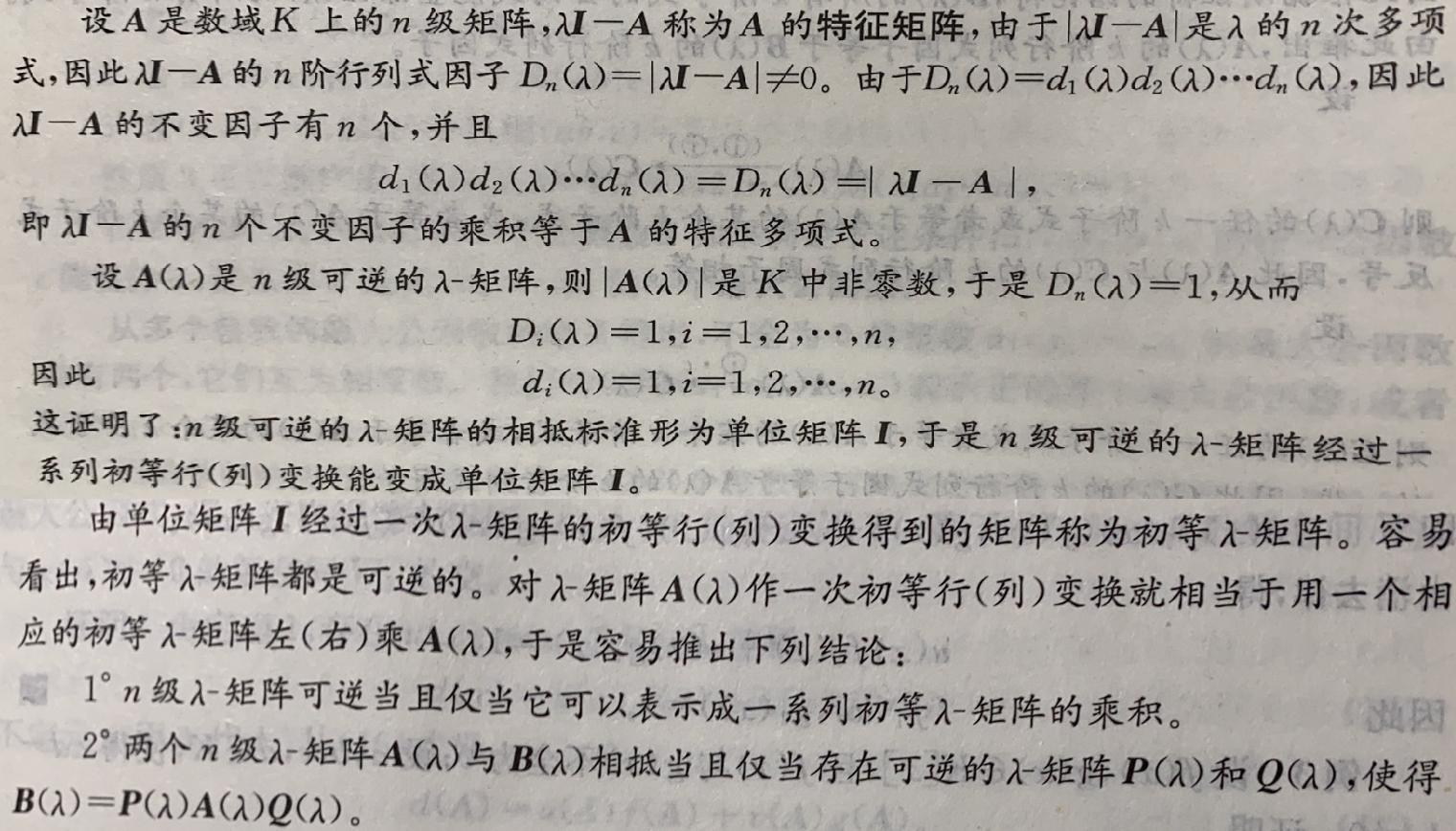二.不可约多项式与唯一分解定理(7.4)1.不可约多项式
(1)定义:(2)性质:

定理9:设$p(x)$是$K[x]$中1个次数大于0的多项式,则下列命题等价:
①$p(x)$是不可约多项式(因式的角度)
②对$∀f(x)∈K[x]$,有$p(x)\,|\,f(x)$或$(p(x),f(x))=1$(与任一多项式的关系的角度)
③在$K[x]$中,从$p(x)\,|\,f(x)g(x)$可推出(整除关系的角度)$p(x)\,|\,f(x)或p(x)\,|\,g(x)$
④在$K[x]$中,$p(x)$不能分解成2个次数较低的多项式的乘积(因式分解的角度)
注:括号中说明的是该命题从哪个角度对不可约多项式进行刻画推论1:在$K[x]$中,如果$p(x)$不可约,且$p(x)\,|\,f_1(x)f_2(x)...f_s(x)$那么$∃j∈\{1,2...s\}$,使得$p(x)\,|\,f_j(x)$
推论2:在$K[x]$中,一次多项式是不可约的
推论3:在$K[x]$中,$f(x)$可约当且仅当$f(x)$可以分解成2个次数较低的多项式的乘积

2.唯一因式分解定理
(1)唯一因式分解定理:

定理10:$K[x]$中任一次数大于0的多项式$f(x)$能够唯一地分解成数域$K$上有限多个不可约多项式的乘积;所谓唯一是指,如果$f(x)$有2个这样的分解式$f(x)=p_1(x)p_2(x)...p_s(x)=q_1(x)q_2(x)...q_t(x)\qquad(1)$那么一定有$s=t$,且适当排列因式的次序后有$p_i(x)\sim q_i(x)\,(i=1,2...s)$(2)标准分解式:3.推广到整数环$Z$上
(1)素数与合数:(2)性质:

定理11:设$1,则下列命题等价
①$p$是素数
②对$∀a∈Z$,都有$p\,|\,a$或$(p,a)=1$
③在$Z$中,从$p\,|\,ab$可推出$p\,|\,a$或$p\,|\,b$
④$p$不能分解成2个较小的正整数的乘积(3)算数基本定理:

定理12:任一大于1的整数$a$都能唯一地分解成有限多个素数的乘积;所谓唯一是指,如果$a$有2个这样的分解式$a=p_1p_2...p_s=q_1q_2...q_t$那么$s=t$,且适当排列因数的次序后,有$p_i=q_i\,(i=1,2...s)$
该定理揭示了整数环$Z$的结构

(4)标准分解式:三.重因式(7.5)1.定义:2.一元多项式的导数:3.重因式的存在性与判定:

定理13:设$K$是数域,在$K[x]$中,如果不可约多项式$p(x)$是$f(x)$的1个$k(k≥1)$重因式,那么$p(x)$是$f'(x)$的1个$k-1$重因式;特别地,$f(x)$的单因式不是$f'(x)$的因式推论1:设$K$是数域,在$K[x]$中,不可约多项式$p(x)$是$f(x)$的1个重因式当且仅当$p(x)$是$f(x)$与$f'(x)$的1个公因式推论2:设$K$是数域,在$K[x]$中,次数大于0的多项式$f(x)$有重因式当且仅当$f(x)$与$f'(x)$有次数大于0的公因式推论3:设$K$是数域,在$K[x]$中,次数大于0的多项式$f(x)$没有重因式当且仅当$f(x)$与$f'(x)$互素推论4:设数域$F$包含数域$K$,对于$K[x]$中次数大于0的多项式$f(x),f(x)$在$K[x]$中没有重因式当且仅当$f(x)$在$F[x]$中没有重因式,即$f(x)$
有无重因式不随数域的扩大而改变展开全文抽象代数
• 借助复数域K上n阶矩阵A的Jordon标准形JA，给出A的一种特殊分解式，讨论矩阵多项式空间研K[JA]={f{JA)f(x)∈K[x]}的基及其子空间的直和分解，进而确定复数域上矩阵多项式空间K[A]的一个关于子空间的直和分解式及其一...
• ## 多项式环

千次阅读 2018-11-22 09:53:47
对于整环RRR，在RRR上定义一个含有为定元xxx的多项式 f(x)=anxn+an−1xn−1+...+a1x+a0,n≥0f(x)=a_nx^n+a_{n-1}x^{n-1}+...+a_1x+a_0,n \ge 0f(x)=an​xn+an−1​xn−1+...+a1​x+a0​,n≥0mmm是使得am≠0a_m \neq0...
1.定义
对于整环$R$，在$R$上定义一个含有为定元$x$的多项式
$f(x)=a_nx^n+a_{n-1}x^{n-1}+...+a_1x+a_0,n \ge 0$$m$是使得$a_m \neq0$的最大的整数，那么定义$deg(f(x)) = m$。$a_m$称为首项系数，默认取$a_m=1$。当$f(x)=0$时，规定$deg(f(x))=-\infty$。
把$R$上全体多项式的集合记作$R[x]$。
定义两个多项式相等如下：
$f(x)=\sum_{i=0}^na_ix^i$$g(x)=\sum_{i=0}^mb_ix^i$$f(x)=g(x)$等价于$m=n$且$a_i=b_i,0\le i\le n$。
2.运算
（1）加法：
$f(x)+g(x) = \sum_{i=0}^M(a_i+b_i)x^i$其中$M=max(n,m)，a_i=0(i\gt n)，b_i=0(i\gt m)$
（2）乘法：
$f(x)\cdot g(x)=\sum_{s=0}^{m+n}(\sum_{i+j=s}a_ib_j)x^s$$R[x]$对于多项式的加法和乘法构成的环称为多项式环。和整数环相比较，多项式环是无限的（因为多项式的$deg$是无限的）。
规定$x^0=1$是$R[x]$中的单位元，逆元$-f(x)=\sum_{i=0}^n(-a_i)x^i$。更为重要的一点，多项式相加没有进位，而整数相加有进位，这是多项式优于整数的一大原因。
3.多项式的带余除法
类比整数的带余除法，理解多项式的带余除法。设$f(x),g(x)\in F[x],g(x) \neq 0$，那么是一定有$q(x),r(x)\in F[x]$，满足
$f(x)=q(x)\cdot g(x)+r(x)$其中$deg(r(x))\lt deg(g(x))$或者$r(x)=0$。可以证明$q(x)，r(x)$是唯一的。当$r(x)=0$时，则称$g(x)$整除$f(x)$。$g(x)$称为$f(x)$的因式，$f(x)$称为$g(x)$的倍式。
如果$d(x)$是$f(x)$的因式同时也是$g(x)$的因式，那么称$d(x)$是$f(x)$和$g(x)$的公因式。如果$f(x)$和$g(x)$的任意一个公因式都是$d(x)$的因式，那么$d(x)$则是最大公因式，记作
$d(x)=gcd(f(x),g(x))$一般情况将$d(x)$的首项系数调整为1，从而唯一化$d(x)$。
类似整数中的组合系数，对于$d(x)$也有$d(x)=u(x)\cdot f(x)+v(x)\cdot g(x)$特殊的，当$f(x)$与$g(x)$互素的时候有$1=u(x)\cdot f(x)+v(x)\cdot g(x)$对于多项式，可以证明也有$gcd(f(x),g(x))=gcd(g(x),r(x)) *$因此也可以进行辗转相除法：
$f(x)=q(x)\cdot g(x) + r_0(x),0<deg(r_0(x))<deg(g(x))$$g(x)=q_1(x)\cdot r_0(x) + r_1(x),0<deg(r_1(x))<deg(r_0(x))$$r_0(x)=q_2(x)\cdot r_1(x)+r_2(x),0<deg(r_2(x))<deg(r_1(x))$$...$$r_{n-2}(x)=q_{n}(x)\cdot r_{n-1}(x)+r_n(x),0<deg(r_n(x))<deg(r_{n-1}(x)$$r_{n-1}(x)=q_{n+1}(x)\cdot r_n(x)$从上述方程可以得到$gcd(r_{n-1}(x),r_n(x))=r_n(x)$，根据*式有$gcd(r_{n-2}(x),r_{n-1}(x))=r_n(x)$，…，因此$gcd(f(x),g(c))=r_n(x)$。
根据上述关系可以通过回溯的方法求得系数多项式。但是和整数的辗转相除法一样，回溯的复杂度太高，于是也有直接计算系数多项式的算法。
令$u_{-2}(x)=1,v_{-2}(x)=0,u_{-1}(x)=0,v_{-1}(x)=1$，算法流程如下：

$f(x)$
$g(x)$
$r(x)$

$u_{-2}(x)=1$
$v_{-2}(x)=0$
$r_{-2}(x)=f(x)$

$u_{-1}(x)=0$
$v_{-1}(x)=1$
$r_{-1}(x)=g(x)$

$u_0(x)=1$
$v_0(x)=-q_0(x)$
$r_0(x)=r_{-2}(x)-q_0(x)\cdot r_{-1}(x)$

…
…
…

$u_{n-1}(x)$
$v_{n-1}(x)$
$r_{n-1}(x)\neq 0$

$u_n(x)$
$v_n(x)$
$r_n(x) = 0$

通过上述表格，我们可以得到如下的关系：
$r_i(x)=r_{i-2}(x)-q_i(x)\cdot r_{i-1}(x)$ $u_i(x)=u_{i-2}(x)-q_i(x)\cdot u_{i-1}(x)$ $v_i(x)=v_{i-2}(x)-q_i(x)\cdot v_{i-1}(x)$ 其中$q_i(x)=\frac{r_{i-2}(x)}{r_{i-1}(x)}$，$deg(r_i(x))<deg(r_{i-1}(x))$。
4.多项式的因式分解
一个多项式$f(x)$，如果不能分解为$deg$更小的多项式之积的形式，那么则说$f(x)$是不可约多项式。当一个多项式是可约多项式的时候，可以证明它分解为不可约多项式的形式是唯一的，即
$f(x)=c\cdot p_1^{r_1}(x)\cdot p_2^{r_2}(x)\cdot ...\cdot p_m^{r_m}(x)$是唯一的。其中$c$是$f(x)$的首项系数，$p_i(x)$是首项系数为1的不可约多项式。上式称为标准分解式。
如果一个多项式$p(x)$满足$p^k(x)\mid f(x) \& p^{k+1}(x)\nmid f(x)$那么则称$p(x)$是$k$重因式。
5.多项式同余
多项式$g(x),h(x),f(x)\in F[x]$，如果满足：$g(x)=h(x) mod f(x)$那么则说$g(x)$和$h(x)$模$f(x)$同余。可以证明，多项式同余等价于$f(x)\mid g(x)-h(x)$等价于$g(x)=k\cdot f(x) + h(x)$等价于$r_1(x)=r_2(x)$其中$g(x)=q_1(x)\cdot f(x)+r_1(x)$$h(x)=q_2(x)\cdot f(x)+r_2(x)$$deg(r_1(x))<deg(f(x))\& deg(r_2(x))<deg(f(x))$可以证明每一个多项式都与唯一一个次数小于$deg(f(x))$的多项式同余。进一步，多项式同余是一个等价关系。所以可以构造多项式的剩余类环。
由多项式$f(x)$生成的理想记作$<f(x)>$，定义商环：$F[x]/<f(x)>=\{[r(x)]|0\le deg(r(x))\lt deg(f(x))\}=\{r_{n-1}x^{n-1}+...+r_1x+r_0|n=deg(f(x)),r_i\in F,0\le i\le n-1\}$可以证明$[r(x)]=r(x)+<f(x)>$
6.模多项式乘法逆元
设多项式$f(x),g(x)\in F[x]$为非零多项式，$g(x)modf(x)$有乘法逆元当且仅当$gcd(g(x),f(x))=1$进一步对于任意$f(x)\in F[x]$,$f(x)=a_nx^n+a_{n-1}x^{n-1}+...+a_1x+a_0$定义其形式微商如下:$f^{'}(x)=n\cdot a_nx^{n-1}+(n-1)\cdot a_{n-1}x^{n-2}+...+a_1$可以证明$f(x)$没有重因式等价于$gcd(f(x),f^{'}(x))=1(f(x)\neq 0)$。
7.余元定理
设$f(x)\in F[x]，\alpha \in F$，用单项式$x-\alpha$除$f(x)$得到的余式是域$F$中的元素$F(\alpha)$。
由上述定理可以推导出：
$(x-\alpha)\mid f(x) \Longleftrightarrow \alpha 是f(x)的根\Rightarrow deg(f(x))=n,则f(x)在F中最多有n个两两不同的根$


展开全文数学
• 本节主要介绍最小多项式与若尔当标准型的相关知识，这是考研中常考的一部分内容，几乎每个院校都有所考察，对于若尔当标准型，请大家先把第八章 矩阵的知识掌握，这样有利于更好的熟悉这一块内容,在真正考试的时候，...c++求矩阵的秩 已知旋转矩阵求角度
• 一.一元多项式的根(7.6) 二.复数域上的不可约多项式(7.6) 三.实数域上的不可约多项式(7.7) 四.实数系多项式的根(7.7)
• 一.n元多项式环 1.nnn元多项式的概念 2.nnn元多项式的运算 3.数域KKK上nnn元多项式K[x1,x2...xn]K[x_1,x_2...x_n]K[x1​,x2​...xn​]的通用性质 4.nnn元多项式函数 ...3.数域KKK上一元多项式的判别 ...
• 全域多项式插值指的是在整个插值区域内形成一个多项式函数作为插值函数。关于多项式插值的基本知识，见“计算基本理论”。 　在单项基插值和牛顿插值形成的表达式中，求该表达式在某一点处的值使用的Horner嵌套...
• 文章目录多项式函数与根多项式函数的有关性质例1多项式函数的k重根例2例3例 4例5例 6参考资料 多项式函数与根 1、多项式函数 定义\large\color{magenta}{\boxed{\color{brown}{定义} }}定义​ 设 f(x)=a0...的表示线性规划 自然语言处理 机器学习 数据挖掘
• 全域多项式插值指的是在整个插值区域内形成一个多项式函数作为插值函数。关于多项式插值的基本知识，见“计算基本理论”。在单项基插值和牛顿插值形成的表达式中，求该表达式在某一点处的值使用的Horner嵌套算法啊...
• 首先将一个矩阵和一个多项式对应起来（矩阵的多项式，矩阵的零化多项式，相似的矩阵对应零化多项式有相同的最小多项式[https://zhidao.baidu.com/question/273308991.html]） 两个相似的矩阵就是同一个线性映射在...
• 寻找零化多项式的一种方法 摘自邱维声《高等代数(下)》Chapter 10.1, Page 270 的最小多项式 分块多角化矩阵线
• ## MATLAB之多项式插值

千次阅读 2020-04-12 09:28:31
函数解析未知，但已知一些列点的函数值。如下表所示，对于n+1个点，我们可以找到一个次数不超过n的插值多项式。 ，称f为x的n次插值多项式。 x0 x1 x2 x3 ...... xn f(x0) f(x1) f(x2) ...matlab 算法
• 文章目录复系数多项式实系数多项式例1例2例 3参考资料 复系数多项式 1、代数基本定理 ∀f(x)∈C[x],\forall f(x) \in C[x],∀f(x)∈C[x], 若 ∂(f(x))≥1,\partial(f(x)) \geq 1,∂(f(x))≥1, 则 f(x)f(x)f(x) 在...线性代数 自然语言处理 数据挖掘 机器学习 深度学习
• 文章目录整系数多项式埃森斯坦判别法例1例2整系数多项式的有理根例 3参考资料 整系数多项式 设 f(x)∈Q[x].f(x) \in \mathbb{Q}[x] .f(x)∈Q[x]. 记 f(x)f(x)f(x) 的系数的公分母为 d,d,d, 则 df(x)d f(x)df(x)为整...自然语言处理 机器学习 数据挖掘 线性代数 color
• 文章目录最大公因式定义辗转相除法依据的原理定理2（最大公因式的表示）互素定理3:互素的充要条件定理4证明推论证明不可约多项式定理5因式分解及唯一性定理标准分解式 最大公因式定义 f(x),g(x)∈P[x],d(x)∈P[x]f...
• 本文使用 Zhihu On VSCode 创作并发布0 前言自动驾驶开发中经常涉及到多项式曲线拟合，本文详细描述了使用最小二乘法进行多项式曲线拟合的数学原理，通过样本集构造范德蒙德矩阵，将一元 N 次多项式非线性回归问题...
• 介绍了极小多项式和友矩阵的相关概念以及基础性质。 极小多项式 多项式 $$p(t)$$ 称为使 $$A\in M_n$$ 零化，如果 $$p(A)=0$$. Cayley-Hamilton 定理保证了：对每个 $$A \in M_n$$, 存在一个 $$n$$ 次的首 1 ...
• Matlab中的数据分析与多项式计算 更新时间：2019·04·01 更新内容： 数据统计分析 函数 函数使用 描述 备注 Max [y,k]=Max(a)/Max(A) 取向量a或矩阵A的最大元素y为序号k对应的值 如果元素为复数则按模取...MATLAB 数据分析 曲线拟合
• 开始补EC PK赛的题目，在此之前决定先完善多项式全家桶的学习任务。 FFT 在O(logN)O(logN)O(logN)的时间完成点值、系数的多项式表示，在O(N)O(N)O(N)的时间完成多项式乘积。 FFT在之前学习过：[多项式全家桶] ...数学
• 文章目录重因式定义定理6推论 1推论 2推论 3小妙招：去除多项式中的重因式定理7（余数定理）定理8定理9 重因式定义 p(x)p(x)p(x)是不可约多项式 pk(x)∣f(x)p^k(x)|f(x)pk(x)∣f(x) pk+1∤f(x)p^{k+1}\nmid f(x)pk+...
• 满秩分解 定义与性质 定义1 满秩分解：对于m×nm×nm \times n的矩阵AAA，假设其秩为rrr，若存在秩同样为rrr两个矩阵：Fm×rFm×rF_{m \times r}（列满秩）和Gr×nGr×nG_{r \times n}（行满秩），使得A=FGA=FGA...线性代数
• ## 多项式长除法

千次阅读 2014-05-02 18:07:00
2019独角兽企业重金招聘Python工程师标准>>> ...
• 使Logistic回归适应多类分类问题的一种流行方法是将多类分类问题分解为多个二元分类问题，并在每个子问题上拟合标准Logistic回归模型。这种类型的技术包括一对一包装和一对一包装模型。 一种替代方法涉及更改逻辑...
• （1）多项式回归方程 当两个变数间的曲线关系很难确定时，可用多项式逼近 ，称多项式回归（polynomial regression）。 最简单的多项式是二次多项式，方程为： 三次多项式方程为： 具有两个弯曲和一个拐点 ...
• ## 高等代数---多项式

千次阅读 多人点赞 2020-08-10 19:34:52
高等代数–多项式 声明： 本篇文章内容主要对《高等代数》第三版第一章内容的总结，复习。 数域 按照所研究的问题，我们常常需要明确规定所考虑的数的范围，在不同的范围内，所得到的结果可能是不同的。 数的概念...
• 本文证明了定理设F是一个特征为P的含P~a个元的有限域.f(x)=f_1(x)~l1…f_k(x)~lk是f(x)在多项式环F[x]中的标准分解式,f_i(x)是最高系数为1、次数为n_i的不可约多项式.那么f(x)有原根的充分必要条件为当p≥3时:k=1...
• 1.多项式时间复杂度定义：问题需要的时间（复杂度）与问题的规模之间是多项式关系。例如，多项式关系形如O(nk)O(n^k)，k为某个常数，n是问题的输入规模。例如，时间复杂度为O(nlog(n))、O(n^3)都是多项式时间复杂度......